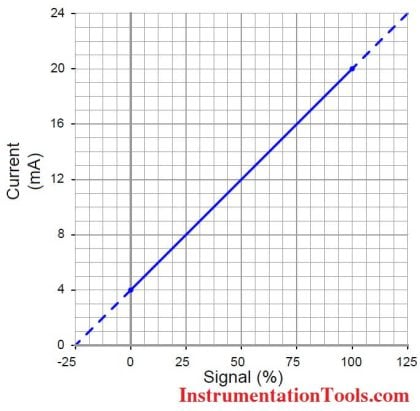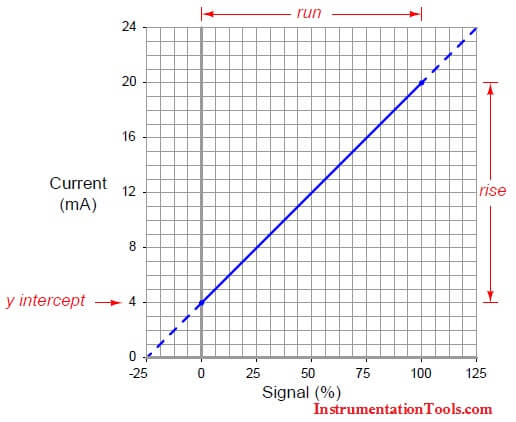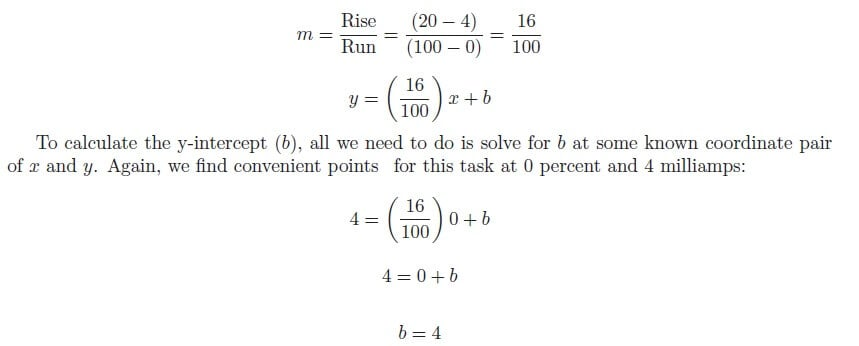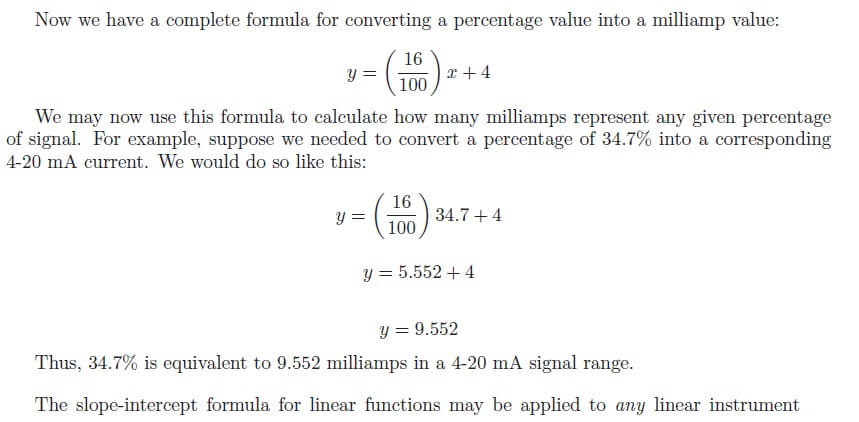# Standard 4 to 20 mA Conversion Formula

A 4 to 20 mA current signal represents a 0 to 100 percent scale. Usually, this scale is linear, as such:

## 4 to 20 mA ConversionBeing a linear function,  we may use the standard slope-intercept linear equation to relate signal percentage to current values:

### y = mx + b

Where,
y = Output from instrument
x = Input  to instrument
m = Slope
b = y-intercept point (i.e.  the live zero of the instrument’s range)

Once we determine suitable values for m and b, we may then use this linear equation to predict any value for y given x, and vice-versa.  This is very useful for predicting the 4-20 mA signal output of a process transmitter, or the expected  stem position of a 4-20 mA controlled valve, or any other correspondence  between  a 4-20 mA signal and some physical  variable.

Before we may use this equation for any practical purpose, we must determine the slope (m) and intercept (b) values appropriate for the instrument we wish to apply  the equation to. Next, we will see some examples  of how to do this.

For the linear function  shown, we may determine the slope value (m)  by dividing  the line’s rise by  its run.   Two  sets  of convenient points we may  use in calculating rise over  run  are  4 and  20 milliamps  (for the rise), and 0 and 100 percent (for the run):Don't Miss Our Updates
Be the first to get exclusive content straight to your email.
We promise not to spam you. You can unsubscribe at any time.

### 13 thoughts on “Standard 4 to 20 mA Conversion Formula”

1.The formula y=mx+b; is a new information.
Thanks for the information.

•Yes, Everyone uses direct formulas for conversion of current to percentage or current to process variable or other related conversations. But these all equations are derived from this standard formula only. Hope my article will be helpful.

•2.I’m trying to solve a liner equation with 4/20mA signal. Can you show me how set up the equation to solve? A value of 17.52mA is measured as the pv of 4/20 mA level loop. What is the URV, if the LRV corresponds to 1.2 meters depth and the PV is known to correspond to 2.9 meters?
thanks Keith

•Hi, URV is around 3.22 meters. Please click here for formula

or

Use the online Instrumentation calculator. Click Here

3.nice information…

4.hiii sir,
we have RECEIVED a flow transmitter make Fns FT 210-1A and it have an analog output of 4-20mA. BUT WE NEED A FLOW TRANSMITTER HAVING RANGE OF (6-20 m3/hr) as per spec. . Is there any idea about correlating or finding the flow rate range with this analog output.

•Hi, Please do re-calibrate your flow transmitter with your required range. Also check the flow transmitter measurable range.

•thanks for your information.
how can i confirm that this FT 210 can have range between 6-20 m3/hr?

5.Check your flow transmitter data sheet or Connect HART communicator to the FT and check Upper Sensor Limit (USL) and Lower Sensor Limit (LSL) which are usually in the mmH20 or related units. Check the Datasheet for the respective pressure values (mmH20) for the 6m3/hr and 20m3/hr and cross check with FT – USL & LSL values. Generally this range (6-20m3/hr) is quite low, so hopefully it will be within the range.

•thank you very much for your kind information

6.Hi,
This formula is not working if value is in negative.
Example: y={20-4/20-(-10)}×5+5

7.